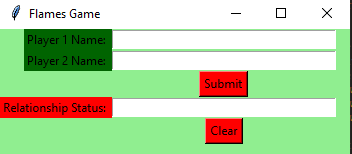# Python | Simple FLAMES game using Tkinter

• Difficulty Level : Hard
• Last Updated : 10 Jun, 2021

Prerequisites:

Python offers multiple options for developing a GUI (Graphical User Interface). Out of all the GUI methods, Tkinter is the most commonly used method. It is a standard Python interface to the Tk GUI toolkit shipped with Python. Python with Tkinter outputs the fastest and easiest way to create GUI applications. Now, it’s up to the imagination or necessity of the developer, what he/she wants to develop using this toolkit.
To create a Tkinter :

• Importing the module – Tkinter
• Create the main window (container)
• Add any number of widgets to the main window.
• Apply the event Trigger on the widgets

The GUI would look like below:Let’s create a GUI version of a simple FLAMES game.FLAMES is a popular game named after the acronym: Friends, Lovers, Affectionate, Marriage, Enemies, Sibling. This game does not accurately predict whether an individual is right for you, but it can be fun to play this with your friends.

Below is the implementation :

## Python3

 `# import all functions from the tkinter``from` `tkinter ``import` `*` `# function for removing common characters``# with their respective occurrences``def` `remove_match_char(list1, list2):` `    ``for` `i ``in` `range``(``len``(list1)) :``        ``for` `j ``in` `range``(``len``(list2)) :` `            ``# if common character is found``            ``# then remove that character``            ``# and return list of concatenated``            ``# list with True Flag``            ``if` `list1[i] ``=``=` `list2[j] :``                ``c ``=` `list1[i]` `                ``# remove character from the list``                ``list1.remove(c)``                ``list2.remove(c)` `                ``# concatenation of two list elements with *``                ``# * is act as border mark here``                ``list3 ``=` `list1 ``+` `[``"*"``] ``+` `list2` `                ``# return the concatenated list with True flag``                ``return` `[list3, ``True``]` `    ``# no common characters is found``    ``# return the concatenated list with False flag``    ``list3 ``=` `list1 ``+` `[``"*"``] ``+` `list2``    ``return` `[list3, ``False``]`  `# function for telling the relationship status``def` `tell_status() :``    ` `    ``# take a 1st player name from Player1_field entry box ``    ``p1 ``=` `Player1_field.get()` `    ``# converted all letters into lower case``    ``p1 ``=` `p1.lower()` `    ``# replace any space with empty string``    ``p1.replace(``" "``, "")` `    ``# make a list of letters or characters``    ``p1_list ``=` `list``(p1)` `    ``# take a 2nd player name from Player2_field entry box``    ``p2 ``=` `Player2_field.get()``    ``p2 ``=` `p2.lower()``    ``p2.replace(``" "``, "")``    ``p2_list ``=` `list``(p2)` `    ``# taking a flag as True initially``    ``proceed ``=` `True``    ` `    ``# keep calling remove_match_char function``    ``# until common characters is found or``    ``# keep looping until proceed flag is True``    ``while` `proceed :` `        ``# function calling and store return value``        ``ret_list ``=` `remove_match_char(p1_list, p2_list)` `        ``# take out concatenated list from return list``        ``con_list ``=` `ret_list[``0``]` `        ``# take out flag value from return list``        ``proceed ``=` `ret_list[``1``]` `        ``# find the index of "*" / border mark``        ``star_index ``=` `con_list.index(``"*"``)` `        ``# list slicing perform``        ` `        ``# all characters before * store in p1_list``        ``p1_list ``=` `con_list[ : star_index]` `        ``# all characters after * store in p2_list``        ``p2_list ``=` `con_list[star_index ``+` `1` `: ]`  `    ``# count total remaining characters``    ``count ``=` `len``(p1_list) ``+` `len``(p2_list)` `    ``# list of FLAMES acronym``    ``result ``=` `[``"Friends"``, ``"Love"``, ``"Affection"``, ``"Marriage"``, ``"Enemy"``, ``"Siblings"``]` `    ``# keep looping until only one item``    ``# is not remaining in the result list``    ``while` `len``(result) > ``1` `:` `        ``# store that index value from``        ``# where we have to perform slicing.``        ``split_index ``=` `(count ``%` `len``(result) ``-` `1``)` `        ``# this steps is done for performing``        ``# anticlock-wise circular fashion counting.``        ``if` `split_index >``=` `0` `:` `            ``# list slicing``            ``right ``=` `result[split_index ``+` `1` `: ]``            ``left ``=` `result[ : split_index]` `            ``# list concatenation``            ``result ``=` `right ``+` `left` `        ``else` `:``            ``result ``=` `result[ : ``len``(result) ``-` `1``]` `    ``# insert method inserting the ``        ``# value in the text entry box.``    ``Status_field.insert(``10``, result[``0``])`  `# Function for clearing the ``# contents of all text entry boxes  ``def` `clear_all() : ``    ``Player1_field.delete(``0``, END)  ``    ``Player2_field.delete(``0``, END)``    ``Status_field.delete(``0``, END)``  ` `    ``# set focus on the Player1_field entry box ``    ``Player1_field.focus_set() `  `# Driver code``if` `__name__ ``=``=` `"__main__"` `:``  ` `    ``# Create a GUI window``    ``root ``=` `Tk()``  ` `    ``# Set the background colour of GUI window``    ``root.configure(background ``=` `'light green'``)``  ` `    ``# Set the configuration of GUI window``    ``root.geometry(``"350x125"``)``  ` `    ``# set the name of tkinter GUI window``    ``root.title(``"Flames Game"``) ``      ` `    ``# Create a Player 1 Name: label``    ``label1 ``=` `Label(root, text ``=` `"Player 1 Name: "``,``                   ``fg ``=` `'black'``, bg ``=` `'dark green'``)``  ` `    ``# Create a Player 2 Name: label``    ``label2 ``=` `Label(root, text ``=` `"Player 2 Name: "``,``                   ``fg ``=` `'black'``, bg ``=` `'dark green'``)``      ` `    ``# Create a Relation Status: label``    ``label3 ``=` `Label(root, text ``=` `"Relationship Status: "``,``                   ``fg ``=` `'black'``, bg ``=` `'red'``)` `    ``# grid method is used for placing ``    ``# the widgets at respective positions ``    ``# in table like structure .``    ``label1.grid(row ``=` `1``, column ``=` `0``, sticky ``=``"E"``) ``    ``label2.grid(row ``=` `2``, column ``=` `0``, sticky ``=``"E"``) ``    ``label3.grid(row ``=` `4``, column ``=` `0``, sticky ``=``"E"``)` `    ``# Create a text entry box ``    ``# for filling or typing the information.``    ``Player1_field ``=` `Entry(root) ``    ``Player2_field ``=` `Entry(root) ``    ``Status_field ``=` `Entry(root)` `    ``# grid method is used for placing ``    ``# the widgets at respective positions ``    ``# in table like structure . ``    ``# ipadx keyword argument set width of entry space . ``    ``Player1_field.grid(row ``=` `1``, column ``=` `1``, ipadx ``=``"50"``) ``    ``Player2_field.grid(row ``=` `2``, column ``=` `1``, ipadx ``=``"50"``) ``    ``Status_field.grid(row ``=` `4``, column ``=` `1``, ipadx ``=``"50"``) ` `    ``# Create a Submit Button and attached ``    ``# to tell_status function ``    ``button1 ``=` `Button(root, text ``=` `"Submit"``, bg ``=` `"red"``, ``                     ``fg ``=` `"black"``, command ``=` `tell_status)``  ` `    ``# Create a Clear Button and attached ``    ``# to clear_all function ``    ``button2 ``=` `Button(root, text ``=` `"Clear"``, bg ``=` `"red"``, ``                     ``fg ``=` `"black"``, command ``=` `clear_all)` `    ``# grid method is used for placing ``    ``# the widgets at respective positions ``    ``# in table like structure . ``    ``button1.grid(row ``=` `3``, column ``=` `1``)``    ``button2.grid(row ``=` `5``, column ``=` `1``)` `    ``# Start the GUI ``    ``root.mainloop()`

Output :

My Personal Notes arrow_drop_up